# Evaluation of Newly Developed Liquid Level Process with PD and PID Controller without Altering Material Characteristics

Evaluation of Newly Developed Liquid Level Process with PD and PID Controller without Altering Material Characteristics
Lecturer, Department of Instrumentation and Control Engineering, Bharathiyar Centenary Memoraial Government Women's Polytechnic College, Ettayapuram- 628902, Thoothukudi District

Professor, Department of Electrical and Electronics Engineering, Hindustan College of Engineering and Technology, Coimbatore – 641 032, TamilNadu

Corresponding Author Email:
sraja034@gmail.com
Page:
218-223
|
DOI:
https://doi.org/10.14447/jnmes.v24i3.a10
1 February 2021
|
Accepted:
30 April 2021
|
Published:
30 September 2021
| Citation

OPEN ACCESS

Abstract:

This article explains the design of fuzzy logic controllers (FLCs) for level processes which is generally used in numerous control operations. The main purpose of the proposed design is to maintain the liquid level in the tank at the desired level. In this paper, the fuzzy logic controller is chosen as the controller for the level process because of its fault tolerance, knowledge representation, expertise, non-linearity, uncertainty, and real-time manipulation. Fuzzy logic controllers have been developed and compared in the Mamdani version. Performance on proportional derivatives (PD) and proportional-integral-derivatives (PID) controllers. Whereas traditional PD and PID controllers are simple, dependable and eliminate steady-state errors, fuzzy logic controllers are rule-based systems that are a logical model of human behavior in processes of the proposed design. The response is provided as follows: The LabVIEW software has been validated. It is used to simulate the proposed system. Comparing error indicators such as PD controller, PID controller, fuzzy logic controller integral absolute error, integral quadratic error, time and absolute error integral, time and quadratic error integral, fuzzy logic controller is observed from the simulation results. increase. It offers better performance than other controllers.

Keywords:

Fuzzy Logic Controllers, Mamdani, Proportional derivative, Proportional integral derivative

1. Introduction

Liquid level monitoring is frequently used in many industrial processes and applications such as water purification, food and beverage processing, impure water management systems, petrochemical processes and others. Controlling these processes requires expertise and in-depth knowledge of the fundamentals and advanced techniques of process control design. Inadequate understanding of the concepts by students who are hired into executive, control engineer, process engineer and management positions in turn can lead to loss of life and property. Commercial application of Proportional Derivative (PD), proportional-integral-derivatives (PID) and Fuzzy Logic Controllers for industrial operations has been going on for several decades. To better understand this work, we give a brief description of PD, PID and FLC as follows. The PD controller is used to increase the stability of the system. The PD controller is capable of predicting future error. of the response, which improves system control. To avoid the effect of abrupt changes in the value of the error signal, the derivative is taken from the output response of the system variable instead of the error signal. Therefore, the "D" mode in the PD is designed to be proportional to the change of the output variable to prevent sudden changes from occurring in the control output due to the sudden change of the error signal. In addition, “D” directly amplifies the process noise. In PD controller there is no feedback, so PID controller is the most widely applied feedback control formula/algorithm. The PID controller has three parameters that must be set. The proportional term (Kp), the integral term (Ti) and the differential term (Td). Figure 1 below shows the effect of the Kp and Ti changes.

In addition, many different controllers have been proposed in different fields , but due to the non-linearity of the system components and substitutions, these evolved feedback controllers are not can effectively control the liquid level and is quite slow on the output feedbacks. . In addition, traditional computer logic is based on two values, one (true) and zero (false), and is not suitable for human decision-making. Therefore, it is often desirable to have a system that behaves like a human. Fuzzy logic uses the entire interval from 0 to 1 and can therefore be used to closely mimic human inference. Fuzzy control is the most suitable system to obtain promising results in case of correct selection of membership and rules . The process of designing a fuzzy logic system can be divided into three stages, namely, fuzzification, fuzzy inference engine and defuzzification. converting crisp value into fuzzy value is called Fuzzification process. Knowledge bases include databases and rule bases used to represent human knowledge, in the form of natural language expressions. Fuzzy inference engine is a way to map input space with output space using fuzzy logic. Defuzzification is the block that converts a fuzzy quantity into a crisp quantity.

## 1.png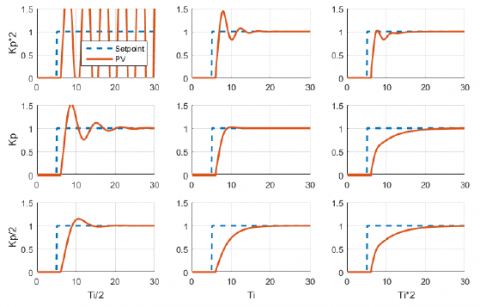Figure 1. Effects of varying Kp and Ti

## 2.png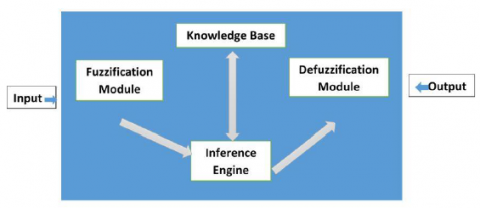Figure 2. Representation of Fuzzy Logic Controller by block diagram

The organization of this paper are as follows: Section 2 presents the existing works in liquid level controller. Proposed process description is discussed in Section 3. Experimental results are evaluated and discussed in Section 4.

2. Existing Works

Literature survey of many studies contain process optimization and control applications have proposed for various industrial areas. Some of these studies are listed in the Table 1 as follows;

Table 1. Existing works

 Authors Proposed Work Kwok et.al., (200) constrained predictive control algorithm based on feedback linearization  Poulsen et.al., (2001) second-order nonlinear system by variable structure controller Sbarbaro and Ortega (2007) To control the multi-tanks systems  Almutairi et al. (2007) Diagrams of sliding mode control for the coupled tanks  Lee and Shin (2009) Conventional PI (proportional-integral) controller based constrained control to liquid level system using  Zhang et al. (2011) non-linear based predictive control  Noel and Pandian (2014) nonlinear liquid level system control by artificial neural network based reinforcement learning method  Sadeghi etal. (2014) Takagi-Sugeno model for a liquid level system by parallel distributed compensation via fuzzy  Singh et al. (2014) reduced flow rate fluctuation for tank in series with PI controller  Zhang et al. (2014) liquid level in a coke fractionation tower PI controller based on predictive functional control system ,  Tao et al. (2014) genetic algorithm for chamber pressure in coke furnace by predictive control based PID design  Zhang and Yang (2014) EGG-data-based estimation of operator functional state by incremental-PID- controlled particle swarm optimization algorithm  Sahu et al. (2015) fuzzy-PID   controller  for automatic generation control of multi-area power system  Gizi et al. (2015) an integrated approach programmable logic controller-fuzzy PID methodology to determine optimal PID parameter in automatic voltage regulator 
3. Fuzzy Logic Controller Development

To design the proposed fuzzy logic controller (FLC), the variable that can represent the dynamic performance of the controlled setting must be selected as the input of the controller. The fuzzy logic controller has two input variables, i.e. error (e) and error rate of change (de), which generates a control signal (cs)..

• Step 1: The variables of input and output are converted into Language variables.
• Step 2: variables of the Language consists of five fuzzy subsets, called as: {Big Negative (BN), Small Negative (SN), Absolute Zero (AZ), Small Positive (SP), Big Positive (BP)}
• Step 3: Every membership function has certain limits, that may be taken to the FLC

## 3.png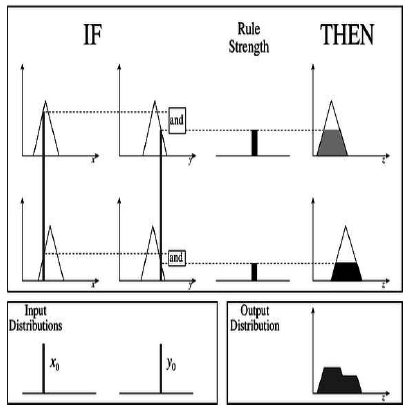Figure 3. Mamdani fuzzy inference

• Step 4: Plotting the fuzzy logic controller rule base The rate of change of the input error (e) and the error combination (de) that change the control signal (cs) for the fuzzy rule base is shown in the table below. 2. A table can be obtained based on 5 fuzzy language variables.

Table 2. Rule base Tabulation

 BP SP AZ SN BN BP BP BP BP SP AZ SP BP SP SP AZ SN AZ BP SP AZ SN BN SN SP AZ SN SN BN BN AZ SN NB BN BN
• Step 5: Block diagram of Mamdani fuzzy inference is shown below for the Selected Mamdani Fuzzy Inference System for the Fuzzy Logic Controller,
• Step 6: Error signal (ES) and differential error signal (DE) are two input variables of fuzzy controller. Errors and differential error signals are handled by a series of conditional statements such as IF the error is "HN" and change the error to "PH" THEN input to the actuator is "ZE".
4. Experimental Setup

In the test setup, the process with the load variable should be a constant constant value and the setpoint value a variable with variable values. A closed loop response for a nonlinear liquid level bath is achieved, for the change of setpoint value, using the proposed Mamdani fuzzy logic controller and PD and PID controllers. To work, the Fuzzy Logic controller with a height of 20 cm from 0 to 500 s and furthermore the height is increased by 10 cm from 500 to 1000 s. To measure performance, error metrics such as integral absolute error (IAE), integral squared error (ISE), integral of time and absolute error (ITAE) and integral of time and squared error (ITSE) are taken into account for consideration..

The procedure of the experimental work can be expressed as follows:

1. 10 liters of water is used to fill the storage tank.
2. The computer based system was manipulated which is provided with all the hard-ware devices required for automatic control of ADC (Analog to Digital Converter), DAC (Digital to Analogue converter), and DLI/O (Digital Logic In / Out).
3. water level has to be set at a certain input value.
4. The steady-state values of water level is recorded by the computer based system which was set automatically.
5. A step change in the set point was carried.
6. System was manipulated to eradicate the error (ISE, ITSE, IAE, ITAE) values in water level (output variable) using Mamdani Fuzzy Logic controllers
7. The level of water versus time were recorded and plotted for the values of the errors (ISE, ITSE, IAE, ITAE).

Fuzzy Logic controller is designed to be compared with PD and PID controllers for liquid level process in Servo- Regulatory operation, Servo Operation and Regulatory Operation. The performance of the controller is evaluated by the error reduction performance index values.

Table 3. Comparison of the Performance index values of the Proposed Fuzzy Logic Controller with PD and PID in Servo Operation

 Performance index values Servo Operation Fuzzy Logic Controller PID Controller PD Controller ISE 1518 1585 1690 ITSE 13165 13962 14670 ITAE 4366 4572 4724 IAE 235.28 243.2 256.2

Operation of the servo for the fuzzy logic controller, PID controller and PD controller operate at a height of 50cm from 0 to 1000s and the height is increased by 50cm from 1000 to 2000s. During this servo operation, the above controller performance index values such as ISE, ITSE, IAE and ITAE are collected and tabulated in Table 3. From this table, it can be seen that the Logic controller fuzzy Product has better performance value than PID and PD Controller and the percentage of performance improvement is shown in Table 4.

Table 4. Percentage of performance improvement by FLC in Servo Operation

 Performa nce index values % of improvement by FLC over PID controller % of improvement by FLC over PD controller ISE 4.413702 11.3307 ITSE 6.053931 11.43183 ITAE 4.718278 8.199725 IAE 3.366202 8.891533

In Figure 4 it shown that it is concluded that, the Fuzzy Logic Controller can produce faster settling response and the same is given.

## 4.png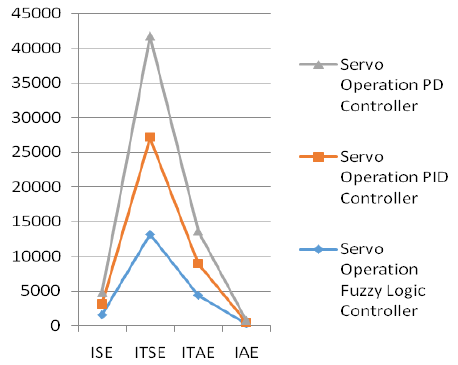Figure 4. Graphical Representation of Servo operation Errors

Table 5. Comparison of the Performance index values of the Proposed Fuzzy Logic Controller with PD and PID in Regulatory Operation

 Performance index values Regulatory Operation Fuzzy Logic Controller PID Controller PD Controller ISE 1965 2062 2160 ITSE 17632 17958 18715 ITAE 5877.3 5982.5 6238.3 IAE 332.3 340.2 352.5

In the regulatory process, the Fuzzy Logic controller and the PI Neuro Tuned controller operate at a height of 60cm from 0 to 3000 s with a load change of +20% after 1000 s. During the throttling operation, the above controller performance index values such as ISE, ITSE, IAE and ITAE are collected and tabulated in Table 5. From the table, it can be seen that the Fuzzy Logic controller generates The performance values are better than that of the PID and PD controller and the performance improvement ratio is shown in Table 6.

Table 6. Percentage of performance improvement by FLC in Regulatory Operation

 Performance index values % of  improvement by FLC over PID controller % of  improvement by FLC over PD controller ISE 4.936387 9.923664 ITSE 1.848911 6.142241 ITAE 1.789938 6.142276 IAE 2.37737 6.078844

In Figure 5 it shown that it is concluded that, the Fuzzy Logic Controller can produce faster settling response and the same is given.

## 5.png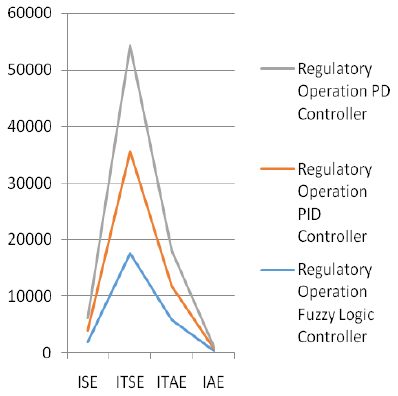Figure 5 Graphical Representation of Regulatory operation Errors

Table 7 Comparison of the Performance index values of the Proposed Fuzzy Logic Controller with PD and PID in Servo– regulatory Operation

 Performance index values Servo - Regulatory Operation Fuzzy Logic Controller PID Controller PD Controller ISE 1318 1372 1418 ITSE 9165 9665 10165 ITAE 4566 4866 5366 IAE 155.28 185.42 205.28

Likewise, in servo regulator operation, the Fuzzy Logic controller and the PI Neuro Tuned controller operate at a height of 50 cm from 0 to 1000 s and a height increase of 50 cm from 1000 s to 2000 s with +20 %load changes every 1000 seconds. During servo operation, the above controller performance index values such as ISE, ITSE, IAE and ITAE are collected and tabulated in Table 7. From this table, it can be seen that Fuzzy Logic controller produces better performance values when compared for PID and PD controllers and the performance improvement ratio is shown in Table 8.

Table 8. Percentage of performance improvement by FLC in Servo Regulatory Operation

 Perform ance index values % of improvement by FLC over PID controller % of improvement by FLC over PD controller ISE 4.097117 7.587253 ITSE 5.455537 10.91107 ITAE 6.570302 17.52081 IAE 19.4101 32.1999

From this, it is concluded that the Fuzzy Logic Controller can produce faster settling response in the servo- regulatory operation and the same is given in Figure 6 .

## 6.png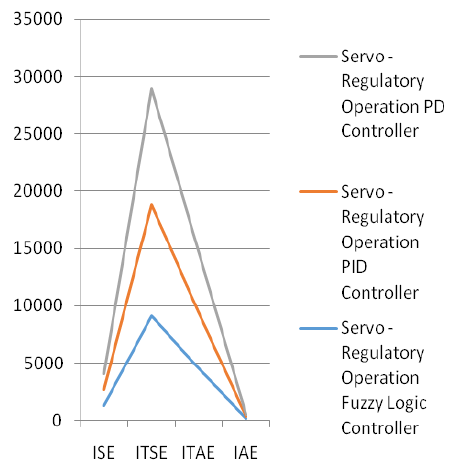Figure 6 Graphical Representation of Servo Regulatory operation Errors

It is seen that the Fuzzy Logic Controller is capable to produce faster response with minimal error, and is also desirable for the liquid level process by the servo, regulatory and servo-regulatory process.

5. Summary and Conclusion

From this study based experimental process it is determined that the Mamdani Fuzzy Logic Controller for the liquid level process provide better performance between the PD and PID controller.The PID controller is better than PD. Thus,the simulation outputs are obtained for this controller by aligning the set point changes, load changes and change in set point with load changes. It clearly shows that the errors for servo, regulatory and servo-regulatory are attenuated substantially.

References

 Zhou, Feng, Hui Peng, Yemei Qin, Xiaoyong Zeng, Wenbiao Xie, and Jun Wu. "RBF-ARX model-based MPC strategies with application to a water tank system." Journal of Process Control 34 (2015): 97-116. https://doi.org/10.1016/j.jprocont.2015.07.010

 Bevrani, Hassan and Pourya Ranjbar Daneshmand "Fuzzy logic-based load-frequency control concerning high penetration of wind turbines."IEEE systems journal 6, no. 1 (2011): 173-180. https://doi.org/10.1109/JSYST.2011.2163028.

 Kwok, K. Ezra, Michael Chong Ping, and Ping Li. "A model-based augmented PID algorithm." Journal of Process Control 10, no. 1 (2000): 9-18. https://doi.org/10.1016/S0959-1524(99)00046-3

 Poulsen, Niels Kjølstad, Basil Kouvaritakis, and Mark Cannon. "Nonlinear constrained predictive control applied to a coupled-tanks apparatus." IEE Proceedings-Control Theory and Applications 148, no. 1 (2001): 17-24. https://doi.org/10.1049/ip-cta:20010231

 Sbarbaro, Daniel, and Romeo Ortega. "Averaging level control of multiple tanks: A passivity based approach." In Proceedings of the 44th IEEE Conference on Decision and Control, pp. 7384-7389. IEEE, 2005. https://doi.org/ 10.1109/CDC.2005.1583353.

 Almutairi, Naif B., and Mohamed Zribi. "Sliding mode control of coupled tanks." Mechatronics 16, no. 7 (2006): 427-441. https://doi.org/10.1016/j.mechatronics.2006.03.001  Lee, Moonyong, and Joonho Shin. "Constrained optimal control of liquid level loop using a conventional proportional-integral controller." Chemical Engineering Communications 196, no. 6 (2009): 729-745. https://doi.org/10.1080/00986440802557393

 Zhang, Ridong, Sheng Wu, and Furong Gao. "Improved PI controller based on predictive functional control for liquid level regulation in a coke fractionation tower." Journal of Process Control 24, no. 3 (2014): 125-132. https://doi.org/10.1016/j.jprocont.2014.01.004.  Noel, Mathew Mithra, and B. Jaganatha Pandian. "Control of a nonlinear liquid level system using a new artificial neural network based reinforcement learning approach." Applied Soft Computing 23 (2014): 444-451. https://doi.org/10.1016/j.asoc.2014.06.037

 Sadeghi, Mokhtar Sha, Behrouz Safarinejadian, and Amir Farughian. "Parallel distributed compensator design of tank level control based on fuzzy Takagi–Sugeno model." Applied soft computing 21 (2014): 280-285. https://doi.org/10.1016/j.asoc.2014.03.024

 Singh, Ajay Pratap, Samrat Mukherjee, and Michael Nikolaou. "Debottlenecking level control for tanks in series." Journal of Process Control 24, no. 3 (2014): 158-171. https://doi.org/10.1016/j.jprocont.2013.12.002

 Zhang, Ridong, Sheng Wu, Renquan Lu, and Furong Gao. "Predictive control optimization based PID control for temperature in an industrial surfactant reactor." Chemometrics and Intelligent Laboratory Systems 135 (2014): 48-62. https://doi.org/10.1016/j.chemolab.2014.03.021

 Tao, Jili, Zaihe Yu, and Yong Zhu. "PFC based PID design using genetic algorithm for chamber pressure in a coke furnace." Chemometrics and Intelligent Laboratory Systems 137 (2014): 155-161. https://doi.org/10.1016/j.chemolab.2014.07.003.

 Zhang, Jianhua, and Shaozeng Yang. "An incremental-PID-controlled particle swarm optimization algorithm for EEG-data-based estimation of operator functional state." Biomedical Signal Processing and Control 14 (2014): 272-284. https://doi.org/10.1016/j.bspc.2014.08.005

 Sahu, Binod Kumar, Swagat Pati, Pradeep Kumar Mohanty, and Sidhartha Panda. "Teaching–learning based optimization algorithm based fuzzy-PID controller for automatic generation control of multi-area power system." Applied Soft Computing 27 (2015): 240-249. https://doi.org/10.1016/j.asoc.2014.11.027

 Al Gizi, Abdullah JH, M. W. Mustafa, Kaml MA Al Zaidi, and Mahmoud KJ Al-Zaidi. "Integrated PLC-fuzzy PID Simulink implemented AVR system." International Journal of Electrical Power & Energy Systems 69 (2015): 313-326. https://doi.org/10.1016/j.ijepes.2015.01.009